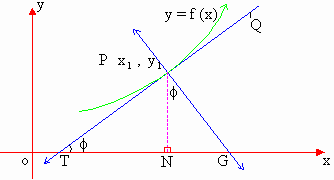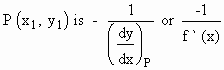Home MonkeyNotes Printable Notes Digital Library Study Guides Study Smart Parents Tips College Planning Test Prep Fun Zone Help / FAQ How to Cite New Title Request

CHAPTER 5 : APPLICATIONS OF THE DERIVATIVE

5.1 Tangent and Normal Lines

The Equation of the Tangent Line to a CurveThe adjoining figure shows the graph of the curve y = f (x), and p is a pointon it. TPQ is the tangent to the curve at P. This is inclined at an angle f to the positive of x-axis.

Then as seen previously, tan f =represents the slope of the tangent line TPQ to the

curve y = f (x) at the point P.Your browser does not support the IFRAME tag.

Thus, the equation of the tangent line to the curve at,The Normal Line is defined as the line which is perpendicular to the tangent at the point of its contact to the curve. Therefore the slope of this normal line at pointTherefore, the equation of the normal line at P to the curve y = f (x) will beIndex

5.1 Tangent And Normal Lines
5.2 Angle Between Two Curves
5.3 Interpretation Of The Sign Of The Derivative
5.4 Locality Increasing Or Decreasing Functions 5.5 Critical Points
5.6 Turning Points
5.7 Extreme Value Theorem
5.8 The Mean-value Theorem
5.9 First Derivative Test For Local Extrema
5.10 Second Derivative Test For Local Extrema
5.11 Stationary Points
5.12 Concavity And Points Of Inflection
5.13 Rate Measure (distance, Velocity And Acceleration)
5.14 Related Rates
5.15 Differentials : Errors And Approximation